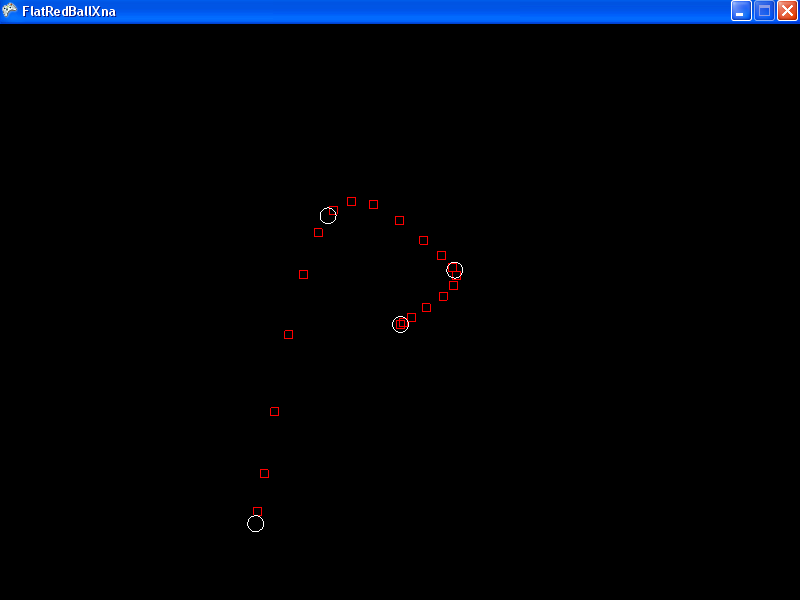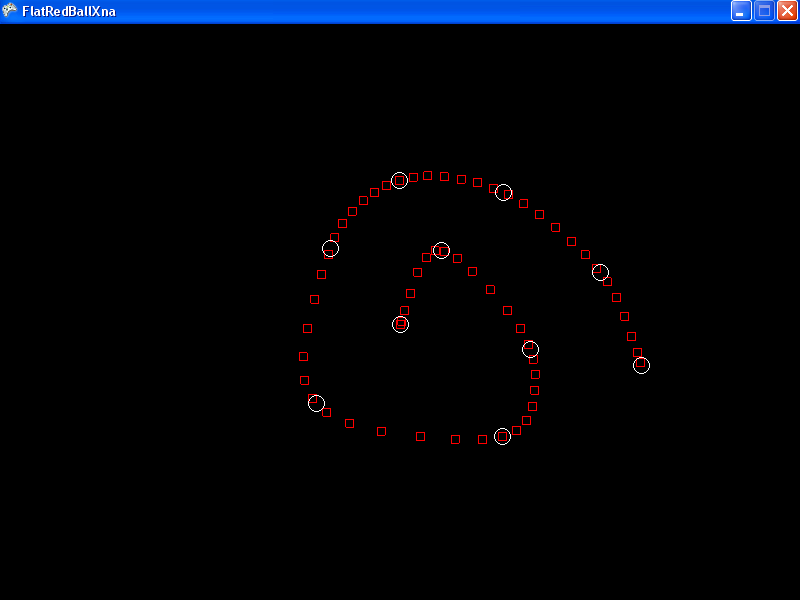# Spline

## Introduction

A Spline is a curved path that you can use to create curved motion. Splines can be created in code or in tools like the SplineEditor. The default file format for a spline is .splx.

## Creating a Spline in code

The following example instantiates a Spline, adds four points, calculates the velocity and acceleration values, then finally makes the Spline visible. Visibility is usually used for tools and debugging.

```using FlatRedBall.Math.Splines;
```

``` // Create a new spline
Spline spline = new Spline();

SplinePoint point = new SplinePoint();
point.Position = new Vector3(0, 0, 0);

// The first point's Time defaults to zero.  Set non-zero
// times for all additional SplinesPoints.
point = new SplinePoint();
point.Position = new Vector3(3, 3, 0);
point.Time = 2;

point = new SplinePoint();
point.Position = new Vector3(-4, 6, 0);
point.Time = 4;

point = new SplinePoint();
point.Position = new Vector3(-8, -11, 0);
point.Time = 6;

// CalculateVelocities must be called before
// CalculateAccelerations is called.
spline.CalculateVelocities();
spline.CalculateAccelerations();

// Let's make it visible so we can see our creation.
spline.Visible = true;
```## Creating a Spline from .splx

The following code creates a Spline by loading a .splx file. First you should add the file to your project. Information on adding files can be found here.

File used:ExampleSpline.splx

```using FlatRedBall.Math.Splines;
```

Add the following to Initialize after initializing FlatRedBall:

``` List<Spline> splineList =

// There's no need to caluclate Velocities!
splineList.CalculateAccelerations();
// We know that this list has 1 Spline, so make that one visible
splineList.Visible = true;
```There are a few things to keep in mind:

• A .splx file can contain multiple Splines. In this case the .splx file only contains one. Splines implement the INameable interface, so you can find Splines in the loaded list by their name if your .splx file contains multiple Splines.
• In this example I added the .splx to my project’s “Content” folder. Therefore, the file name is prepended with “Content\”. Make sure to modify this according to where you place your file.
• .splx files store Velocities, so you do not need to call CalculateVelocities. In fact, it is possible for .splx files to store velocities that have been modified if the tool that has created the .splx supports handles. To preserve the original content, you should only call CalculateAccelerations after the Spline is loaded.

## Positioning objects using a Spline

Objects can be easily positioned using a Spline. The GetPositionAtTime method returns a Vector3. It takes a time argument which is the amount of time from the beginning of the Spline.

The following code positions a Sprite according to how much time has passed since the user has pressed the space bar. This code example also skips over the steps to create a valid Spline and Sprite.

Add the following at class scope:

```double mTimeSplineMovementStarted = 0;
```

```// This code assumes that mySprite is a valid Sprite and mySpline is a valid Spline
if(InputManager.Keyboard.KeyPushed(Keys.Space))
{
mTimeSplineMovementStarted = TimeManager.CurrentTime;
}

mySprite.Position = mySpline.GetPositionAtTime(
TimeManager.SecondsSince(mTimeSplineMovementStarted));
```

## Splines are ILists

Even though Splines provide a variety of methods and properties to treat the Spline as a single object, the Spline object also implements the IList<SplinePoint> interface. That means that you can directly work with a Spline as a set of points.

For example, to move the SplinePoint at index 2 to the right by 4 units, you would write:

```mySpline.X += 2;
```

Of course, if you ever make changes to a Spline like moving its points you will also want to recalculate velocities and accelerations:

```mySpline.CalculateVelocities();
mySpline.CalculateAccelerations();
```

## Spline Members

Did this article leave any questions unanswered? Post any question in our forums for a rapid response.